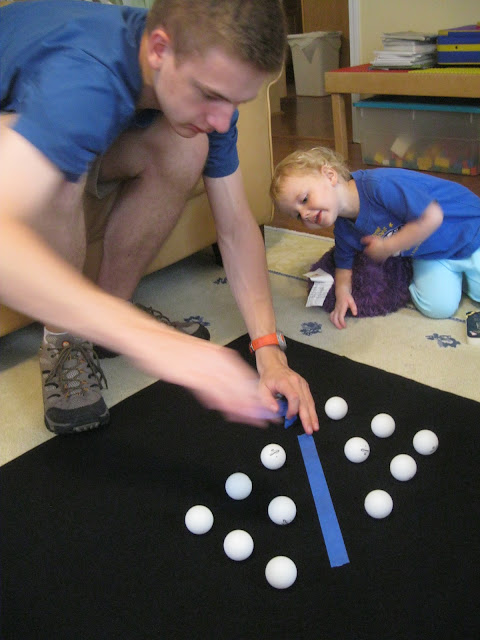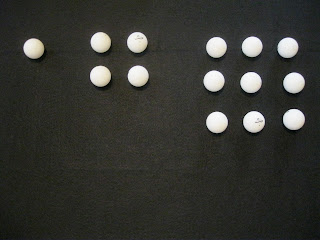## Monday, September 3, 2012

### Montessori Math: Square and Triangular NumbersWe used black felt, ping-pong balls and painter's tape, but you could just use Cheerios on paper, too.

One of the features of Montessori math is the connection between abstract mathematical ideas and hands-on demonstrations of those ideas.  But you don't need expensive equipment to do this.  Here's an example using nothing but ping-pong balls.  (This is my husband's idea of a fun family activity on the long holiday weekend!  It's not a standard Montessori work, but shows what you can do to pass a love of math on to your kids.)

Some numbers correspond to particular shapes.  The simplest of these are the square numbers, such as:

• 1x1 = 1
• 2x2 = 4
• 3x3 = 9
• 4x4 = 16You can show the squared numbers: 1 squared, 2 squared, and 3 squared.

You can show this by lining up squares made from the right numbers of balls.  But it's even possible to use these ping-pong balls to show more complicated algebraic ideas.  For instance, what happens if we take the difference of consecutive squares (the squares of two numbers in a row), like this?

• 2x2 - 1x1 = 3
• 3x3 - 2x2 = 5
• 4x4 - 3x3 = 7

This pattern suggests that the difference between the square of a number and the next biggest square is just twice that number plus 1.  To actually prove it takes algebra or........ ping-pong balls!This is 4 squared minus 3 squared. You could express it algebraically as n + 1 squared minus n squared.
Square numbers are usually covered in school, but who's ever heard of triangular numbers?  They are:

• 1
• 1 + 2 = 3
• 1 + 2+3 = 6
• 1 + 2 + 3 + 4 = 10

and so on.  If you lay out the corresponding number of ping-pong balls, you can see that these numbers form triangles.

Are we having fun yet?!?

If you think THAT's cool, now suppose you want to add up all of the numbers from 1 to 100.  It turns out that this is just equal to 100 x 101 divided by 2.  And this formula holds for any triangular number - just take the last number in the sum, add one, multiply these two numbers together, and divide by 2.  Sure beats adding them all up!  But why does this formula work?  The easiest way to see this is just using the ping-pong balls again!I swear I don't have any stock in the ping-pong ball company.....

I don't know about you, but this makes for interesting dinner conversations and musings.  I think it also makes math seem fun and approachable--maybe (gasp) something even to be enjoyed!She was just waiting for him to make something interesting, like a ducky!The power of the lowly ping-pong ball......